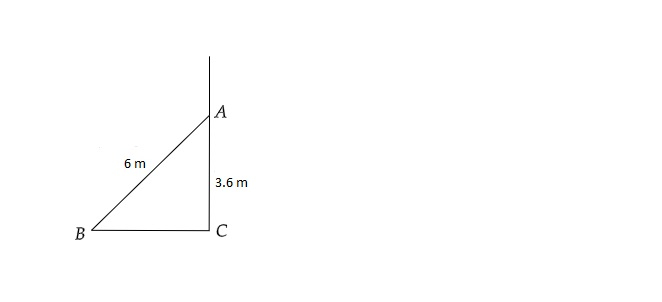# A $6 \mathrm{~m}$ long ladder leans on a wall to reach the height of $3.6 \mathrm{~m}$ on the wall. Find the distance of the lower end of the ladder from the wall.

Given:

A $6 \mathrm{~m}$ long ladder leans on a wall to reach the height of $3.6 \mathrm{~m}$ on the wall.

To do:

We have to find the distance of the lower end of the ladder from the wall.

Solution:Let $AB$ be the ladder and $AC$ be the wall.

$BC$ is be the distance between the wall and the foot of the ladder.

Therefore,

$AB=6\ m$

$AC=3.6\ m$

In $\vartriangle ABC$, using Pythagoras theorem,

$AB^2=AC^2+BC^2$

$\Rightarrow ( 6)^2=( 3.6)^2+BC^2$

$\Rightarrow 36=12.96+BC^2$

$\Rightarrow BC^2=36-12.96$

$\Rightarrow BC^2=23.04$

$\Rightarrow BC=\sqrt{23.04}$

$\Rightarrow BC=4.8\ m$

The distance between the wall and the foot of the ladder is $4.8\ m$.

Updated on: 10-Oct-2022

23 Views